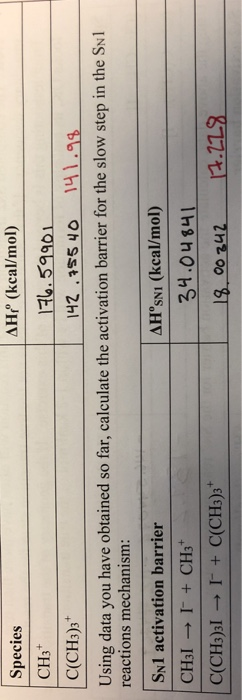# How would I calculate an SN1 activation Barrier when I am given the enthalpies of formation?

how would I calculate an SN1 activation Barrier when I am given the enthalpies of formation?AHP (kcaV/mol) Species CH3 C(CH3)3 Using data you have obtained so far, calculate the activation barrier for the slow step in the Swl 142 55 4o 141.99 reactions mechanism: SN1 activation barrier ΔHoSNI (kcal/mol) 34.04 841 C(CH3)3l I+ C(CH3)3 00 242 4.119

Answer: It is easy with the provided data. The slow step which is otherwise known as the rate-determining step in an SN1 reaction is the formation of carbocation as indicated in the question. Hence, the associated activation energy barrier can easily be calculated by subtracting the given standard change in enthalpy of CH3I (34.04841 kcal/mol) and C(CH3)3I (17.228 kcal/mol) from the standard enthalpy of formation of CH3+ (176.59901 kcal/mol) and C(CH3)3+ (141.98 kcal/mol). Hence, the 142.5506 kcal/mol is the activation barrier associated with the formation of CH3+ from CH3I and 124.752 kcal/mol is the activation barrier associated with the formation of C(CH3)3+ from C(CH3)3I.

#### Earn Coin

Coins can be redeemed for fabulous gifts.